# Similar Triangles and An Intro. to Trigonometry

#### Lesson Overview

Last chapter we introduced the similarity transformation and this chapter we will apply it more specifically to triangles. Similarity between triangles is the basis of trigonometry, which literally means triangle measure. As noted in Numbers lesson 11, the trigonometric functions can be thought of as ratios of the side lengths in right triangles. Please review the informative paragraph and table of special trigonometric values given there.

### Similar Triangles

 If three sides of a triangle are proportional to the three sides of another triangle, then the triangles are similar (SSS Similarity Theorem).

 If the angles (two implies three) of two triangles are equal, then the triangles are similar (AA Similarity Theorem).
Note: this applies not only to ASA, AAS=SAA, but also to AAA situations.

 If two sides of a triangle are proportional to two sides of another triangle and the included angles are congruent, then the triangles are similar (SAS Similarity Theorem).

Thus remains the SSA (ASS) case, which remains ambiguous unless HL or SsA occurs.

 Lines parallel to a side of a triangle intersect the other two sides at nonvertices, if and only if the two sides are split into proportional segments.

Since this theorem is given as an if and only if, it goes both ways. Thus the textbook has both a theorem and its converse.

### Geometric Mean

We introduced the geometric mean somewhat in the last chapter and somewhat in statistics. Please review what we have there. The geometric mean is typically first encountered in a proportion when the means are equal, as in 8/w=w/4. Here w2=32 and square rooting both sides gives an answer. However, in general, there may be n nth geometric means. We thus cannot be sure of the sign of w above.

The geometric mean is developed here because of its application to right triangles and the way the altitude to the hypotenuse divides the triangle into similar triangles. Assume you have two of the three terms in a geometric sequence, such as 2, ?, 50. In other words, you want some number g, such that 2/g=g/50, or 2•50=100=g2, so obviously, g=10 or perhaps g=-10. Often the positive geometric mean is required and will be so specified.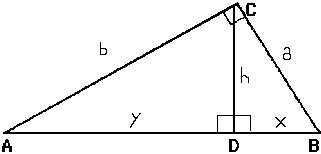The use of lower case letters a, b, and c for the sides of a triangle is a common convention dating back to Euler which we will adhere to. a refers either to the set of points composing the side or the length of the side, depending on context. The angle opposite side a is A, the angle opposite side b is B, and the angle opposite side c is C. If it is a right triangle, C will be right so c will be the [length of the] hypotenuse. Given the right triangle ABC with height h (CD) to the hypotenuse, h=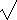(xy), whereas a=(cx), and b=(cy). Here x+y=c (BD + AD = AB), and y is the leg of a similar triangle with hypotenuse b, and x is the leg of a similar triangle with hypotenuse a.

 The altitude of a triangle is the geometric mean of the segments of the hypotenuse that it divides. Each leg would also be a geometric mean of the hypotenuse and the adjacent segment of the hypotenuse.

### Special Triangles, Side Length Ratios, and Trigonometry

An isosceles right triangle (45°–45°–90°) is a very special triangle. Its side lengths form a very special ratio which must be memorized. Specifically, if the legs are both of length x, then the hypotenuse is of length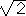x by the pythagorean theorem. This ratio of 1/=/2 or about 0.707 must become familiar.

Similarly, the 30°–60°–90° triangle must be memorized, somehow. One way is to start with an equilateral triangle, bisect one angle which also bisects the side opposite, and consider the resulting congruent triangles. Obviously, two congruent 30°–60°–90° triangles are formed. Again, by the pythagorean theorem, the side length ratios can be found to be 1: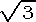:2. By the AA Similarity Theorem, any triangle with these angles has these exact side length ratios.

In right triangles, the six side length ratios are proportional to the angles and named as follows.

 sin A = opposite leg ÷ hypotenuse           csc A = hypotenuse ÷ opposite leg cos A = adjacent leg ÷ hypotenuse           sec A = hypotenuse ÷ adjacent leg tan A = opposite leg ÷ adjacent leg            cot A = adjacent leg ÷ opposite leg

Various mnemonics are commonly employed to assist in the recall of these ratios. SOH-CAH-TOA, with some apocryphal reference to a so named Indian chief is common: S=Sine, O=Opposite, H=Hypotenuse, C=Cosine, A=Adjacent, T=Tangent. Oh Hech Another Hour Of Algebra is another similar mnemonic. Another one only recently brought to my attention is: OHAHOAAO, which is short for Oscar Had A Hand On Alice's Arch Once, which gives, in order, the ratios for Sine, Cosine, Tangent, and Cotangent. Note how the right hand column reciprocates the left hand column. For this reason, the left hand column are considered the primary trigonometric functions and the right hand column secondary. Most calculators only have the primary trig functions.

Consider a right triangle with c=1, one vertex at the origin, one side along the x-axis, and the right angle formed by a perpendicular to the x-axis, thus the hypotenuse lies in quadrant I. In this case we often label one leg y and the other leg x. Consider what happens as we let the angle at the origin change. As the angle increases, the side opposite increases. Since we fixed c=1, that vertex traces out a portion of a unit circle. The sine function describes how this side changes with angle, starting at 0 for 0° and increasing monotonically to 1 for 90° (of course at 0° and 90° it really isn't a triangle). Similarly, the cosine function, which describes how the adjacent side varies, starts out at 1 for 0° and is always decreasing to 0 for 90°.

Tangent is not only the ratio of the opposite side to the adjacent side, but can also be written as sine over cosine. If cosine equals 0, we have a problem, division by zero, so the tangent of 90° is undefined. Large tables of trig functions were commonplace before calculators became ubiquitous. Below is a small table in 5° increments between 0° and 90°. Notice how the cosine values are the same as the sine values of the complementary angle. Tangent and cotangent are similarly related. A later lesson will extend these trig functions past a right angle, into negative angles, graph them, and reintroduce the unit circle.

Angle
(degrees)
Angle
Sine           Cosine       Tangent   Cotangent     Secant      Cosecant
0 0 .00001.0000 .0000NAN 1.0000Infinite
5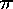/36 .0872 .9962 .087511.4301 1.0038 11.4737
10/18 .1736 .9848 .1763 5.6713 1.0154 5.7588
15/12 .2588 .9659 .2679 3.7321 1.0353 3.8637
20/9 .3420 .9397 .3640 2.7475 1.0642 2.9238
25 5/36 .4226 .9063 .4663 2.1445 1.1034 2.3662
30/6 .5000 .8660 .5774 1.7321 1.1547 2.0000
35 7/36 .5736 .8192 .7002 1.4281 1.2208 1.7434
40 2/9 .6428 .7660 .8391 1.1918 1.3054 1.5557
45/4 .7071 .7071 1.0000 1.0000 1.4142 1.4142
50 5/18 .7660 .6428 1.1918 .8391 1.5557 1.3054
55 11/36 .8192 .5736 1.4281 .7002 1.7434 1.2208
60/3 .8660 .5000 1.7321 .5774 2.0000 1.1547
65 13/36 .9063 .4226 2.1445 .4663 2.3662 1.1034
70 7/18 .9397 .3420 2.7475 .3640 2.9238 1.0642
75 5/12 .9659 .2588 3.7321 .2679 3.8637 1.0353
80 4/9 .9848 .1736 5.6713 .1763 5.7588 1.0154
85 17/36 .9962 .087211.4301 .0875 11.4737 1.0038
90/2 1.0000 .0000 NAN .0000Infinite 1.0000

Note especially NAN for tan 90°=cot 0°. NAN is FORTRANese for Not A Number. It may look like it approaches positive infinity, but since it will approach negative when approaching 90° from above, and since the two limits do not agree, we say it is not a number or undefined.

Example: Consider a flagpole, set precisely perpendicular to the ground, which casts a shadow 11.3355m long and the angle from the ground up at the tip of the shadow to the tip of the pole is 34.5543°. What is the height of the flagpole to correct significance? Solution: Tangent is the ratio of the side opposite the given angle to the side adjacent, thus tan 34.5543°=h/11.3355m. Solving for h we obtain h=11.3355m•tan 34.5543°=7.80650m. If you obtained a negative answer, change your calculator to degrees mode!

The area of any triangle can be found by splitting it into two right triangles.

 Area of a triangle = (1/2)ab•sin C, where C is the angle between sides a and b.

### Vectors, Vector Products, Vector Spaces

Math and physics often classify objects by dimensionality, with scalars (magnitude only) and vectors (magnitude plus direction) occupying the bottom rungs. A classic physics example involves speed (a scalar) and velocity (a vector)–speed tells how fast an object is going, but not in what direction.

One way to develop vectors uses the cartesian coordinate system and ordered pairs or triples. A vector is then defined by the directed line segment from the origin to the given point. The end at the origin is the tail, whereas the other end is the head and often an arrowhead it put there. This gives a certain direction between [0°,360°) and a certain magnitude or length which can be calculated via the distance formula. The vector represented by the directed line segment between (0,0) and (3,4) is the same as the one between (1,1) and (4,5), however, since these have the same magnitude and direction. We often say we can move the vector around as long as we don't change the direction it points or its length. We often break vectors down into x, y, and z components, especially using sines and cosines for the y and x components. Unit vectors, vectors of length 1, especially in the x, y, and z directions are also utilized. There are special names for these: i=(1,0,0), j=(0,1,0), and k=(0,0,1). A unit vector in another direction, such as v=(1,1,1) can be obtained by dividing this vector by its length designated by |v|: (1,1,1)/, where it is understood that the scalar applies to all components of the ordered triple.

Vectors are said to be added head to tail, or if given in component form, via the components. Thus the sum of the vectors (x1, y1, z1) and (x2, y2, z2) is the vector: (x1+x2, y1+y2, z1+z2). Pictorally, the sum is the diagonal of the parallelogram formed by adding them in either order. [make diagram]

The direction of a 2-D vector can be obtained from its x and y components. Specifically, the tangent of the angle relative to the x-axis is equal to y/x. Your calculator has a special key tan-1, better known as atan, to find this arctangent or inverse function. DO NOT confuse this with reciprocal. (It must become clear to you by context whether arctangent or cotangent is meant.) Some adjustment of the calculated value will become necessary since results for quadrants I and IV are assumed. Since our textbook restricts angles to between 0° and 180°, we will defer this into another lesson.

The scalar or dot product of the vectors (x1, y1, z1) and (x2, y2, z2) is the scalar quantity: x1x2+ y1y2+ z1z2. The vector or cross product of two vectors is a more complicated entity which we will leave for a later lesson. Modern physics and mathematics is firmly based on the algebra of vector spaces.